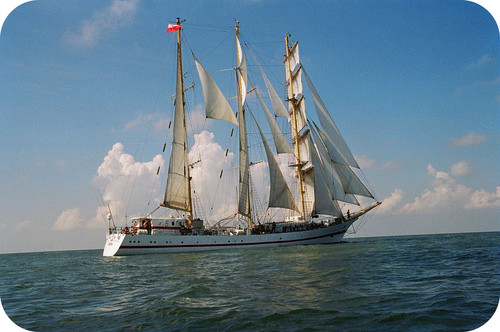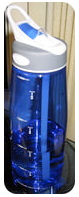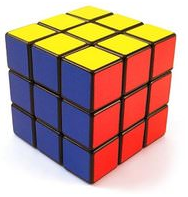# 3.4: Length and Volume

$$\newcommand{\vecs}{\overset { \rightharpoonup} {\mathbf{#1}} }$$ $$\newcommand{\vecd}{\overset{-\!-\!\rightharpoonup}{\vphantom{a}\smash {#1}}}$$$$\newcommand{\id}{\mathrm{id}}$$ $$\newcommand{\Span}{\mathrm{span}}$$ $$\newcommand{\kernel}{\mathrm{null}\,}$$ $$\newcommand{\range}{\mathrm{range}\,}$$ $$\newcommand{\RealPart}{\mathrm{Re}}$$ $$\newcommand{\ImaginaryPart}{\mathrm{Im}}$$ $$\newcommand{\Argument}{\mathrm{Arg}}$$ $$\newcommand{\norm}{\| #1 \|}$$ $$\newcommand{\inner}{\langle #1, #2 \rangle}$$ $$\newcommand{\Span}{\mathrm{span}}$$ $$\newcommand{\id}{\mathrm{id}}$$ $$\newcommand{\Span}{\mathrm{span}}$$ $$\newcommand{\kernel}{\mathrm{null}\,}$$ $$\newcommand{\range}{\mathrm{range}\,}$$ $$\newcommand{\RealPart}{\mathrm{Re}}$$ $$\newcommand{\ImaginaryPart}{\mathrm{Im}}$$ $$\newcommand{\Argument}{\mathrm{Arg}}$$ $$\newcommand{\norm}{\| #1 \|}$$ $$\newcommand{\inner}{\langle #1, #2 \rangle}$$ $$\newcommand{\Span}{\mathrm{span}}$$$$\newcommand{\AA}{\unicode[.8,0]{x212B}}$$Figure $$\PageIndex{1}$$ (Credit: User:Żeglarz/Wikimedia Commons; Source: http://commons.wikimedia.org/wiki/File:Prawa_burta_ORP_Iskra.JPG(opens in new window); License: Public Domain)

### How were sailors able to measure the depths of seas?

Back in the days before all the electronic gadgets for measuring depth and locating undersea objects existed, the "fathom" was the unit of measurement for depth. A rope was knotted every six feet and the end was dropped over the side of the ship. You could tell how deep the water was by how many knots went under the water before the rope hit bottom. Today we just turn on an instrument and read the depth to a high level of accuracy.

## Length and Volume

Length is the measurement of the extent of something along its greatest dimension. The SI basic unit of length, or linear measure, is the meter $$\left( \text{m} \right)$$. All measurements of length may be made in meters, though the prefixes listed in various tables will often be more convenient. The width of a room may be expressed as about 5 meters $$\left( \text{m} \right)$$, whereas a large distance, such as the distance between New York City and Chicago, is better expressed as 1150 kilometers $$\left( \text{km} \right)$$. Very small distances can be expressed in units such as the millimeter or the micrometer. The width of a typical human hair is about 20 micrometers $$\left( \mu \text{m} \right)$$.

Volume is the amount of space occupied by a sample of matter. The volume of a regular object can be calculated by multiplying its length by its width and height. Since each of those is a linear measurement, we say that units of volume are derived from units of length. The SI unit of volume is the cubic meter $$\left( \text{m}^3 \right)$$, which is the volume occupied by a cube that measures $$1 \: \text{m}$$ on each side. This very large volume is not convenient for typical use in a chemistry laboratory. A liter $$\left( \text{L} \right)$$ is the volume of a cube that measures $$10 \: \text{cm}$$ $$\left( 1 \: \text{dm} \right)$$ on each side. A liter is thus equal to both $$1000 \: \text{cm}^3$$ $$\left( 10 \: \text{cm} \times 10 \: \text{cm} \times 10 \: \text{cm} \right)$$, and to $$1 \: \text{dm}^3$$. A smaller unit of volume that is commonly used is the milliliter ($$\text{mL}$$—note the capital $$\text{L}$$, which is a standard practice). A milliliter is the volume of a cube that measures $$1 \: \text{cm}$$ on each side. Therefore, a milliliter is equal to a cubic centimeter $$\left( \text{cm}^3 \right)$$. There are $$1000 \: \text{mL}$$ in $$1 \: \text{L}$$, which is the same as saying that there are $$1000 \: \text{cm}^3$$ in $$1 \: \text{dm}^3$$.Figure $$\PageIndex{2}$$: A typical water bottle is 1 liter in volume. (Credit: User:Kenyon/Wikimedia Commons; Source: http://commons.wikimedia.org/wiki/File:CamelBak_water_bottle.jpg(opens in new window); License: Public Domain)Figure $$\PageIndex{3}$$: This Rubik's cube is $$5.7 \: \text{cm}$$ on each side and has a volume of $$185.2 \: \text{cm}^3$$ or $$185.2 \: \text{mL}$$. (Credit: Bram Van Damme (Flickr: Bramus!); Source: http://www.flickr.com/photos/bramus/3249196137/(opens in new window); License: CC ​​​​​​​by 2.0(opens in new window))Figure $$\PageIndex{4}$$: A graduated cylinder is often used to measure volume in the laboratory, and comes in a variety of sizes. (Credit: User:Darrien/Wikipedia; Source: http://commons.wikimedia.org/wiki/File:Graduated_cylinder.jpg(opens in new window); License: Public Domain)

## Summary

• Length is the measurement of the extent of something along its greatest dimension.
• Volume is the amount of space occupied by a sample of matter.
• Volume can be determined by knowing the length of each side of an item.

## Review

1. Define length.
2. Define volume.
3. An object measures 6.2 cm×13.7 cm×26.9 cm6.2 cm×13.7 cm×26.9 cm. Which value is the length of the object?
4. How big is a mL?

This page titled 3.4: Length and Volume is shared under a CK-12 license and was authored, remixed, and/or curated by CK-12 Foundation via source content that was edited to the style and standards of the LibreTexts platform; a detailed edit history is available upon request.